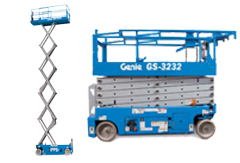###### SCISSOR LIFT###### Specification

Electric Scissor Lifts

GS-1530

Working Height = 6.57 m

Width = .76 m

Lift Capacity = 272 kg

GS-1930

Working Height = 7.79 m

Width = .76 m

Lift Capacity = 227 kg

GS-2032

Working Height = 8.10 m

Width = .81 m

Lift Capacity = 363 kg

GS-2632

Working Height = 9.92 m

Width = .81 m

Lift Capacity = 227 kg

GS-3232

Working Height = 11.75 m

Width = .81 m

Lift Capacity = 227 kg

GS-2046

Working Height = 8.10 m

Width = 1.17 m

Lift Capacity = 544 kg

GS-2646

Working Height = 9.92 m

Width = 1.17 m

Lift Capacity = 454 kg

GS-3246

Working Height = 11.75 m

Width = 1.17 m

Lift Capacity = 318 kg

GS-2669 DC

Working Height = 9.75 m

Width = 1.75 m

Lift Capacity = 680 kg

GS-3369 DC

Working Height = 11.90 m

Width = 1.75 m

Lift Capacity = 454 kg

GS-4069 DC

Working Height = 14.02 m

Width = 1.75 m

Lift Capacity = 363 kg

Rough Terrain Scissor Lifts

GS-2669 RT

Working Height = 9.75 m

Width = 1.75 m

Lift Capacity = 680 kg

GS-3369 RT

Working Height = 11.90 m

Width = 1.75 m

Lift Capacity = 454 kg

GS-4069 RT

Working Height = 14.02 m

Width = 1.75 m

Lift Capacity = 363 kg

GS-3384 RT

Working Height = 12.06 m

Width = 2.13 m

Lift Capacity = 1,134 kg

GS-3390 RT

Working Height = 12.06 m

Width = 2.29 m

Lift Capacity = 1,134 kg

GS-4390 RT

Working Height = 15.11 m

Width = 2.29 m

Lift Capacity = 680 kg

GS-5390 RT

Working Height = 18.15 m

Width = 2.29 m

Lift Capacity = 680 kg

###### Information

Genie self-propelled electric scissor lifts are the industry solution for increasing worksite productivity. Ideal for maneuvering in tight spaces, they are excellent for indoor and outdoor construction, maintenance and installation applications with firm, level surfaces. Characterized by low noise levels, these lifts provide excellent capacity and generous platform workspace.